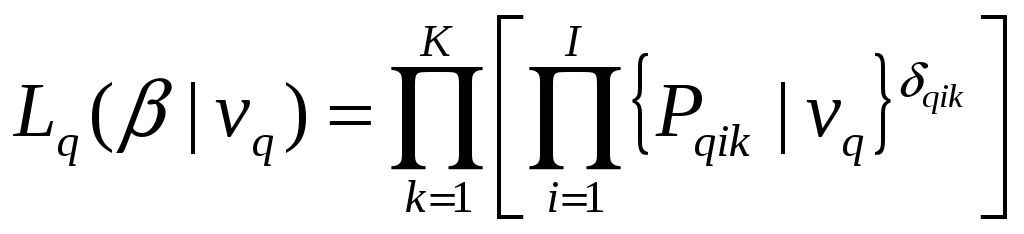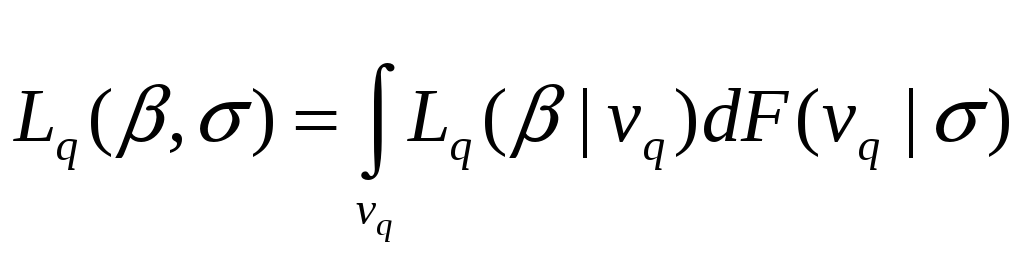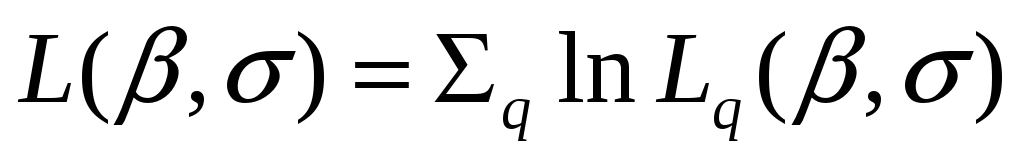# An Analysis of Bicycle Route Choice Preferences in Texas, U. S

 The parameters to be estimated in the model of Equation (3) are the β andvectors. To develop the likelihood function for parameter estimation, we need the probability of each individual's sequence of observed SP choices. Conditional on, the likelihood function for individual q’s observed sequence of choices is:, (4) whereis a dummy variable taking the value of 1 if the qth individual chooses the ith route in the kth occasion, and 0 otherwise. The unconditional likelihood function for individual q’s observed set of choices is:(5) The log-likelihood function is. We apply quasi-Monte Carlo simulation techniques to approximate the integrals in the likelihood function and maximize the logarithm of the resulting simulated likelihood function across all individuals with respect to the parametersand. Under rather weak regularity conditions, the maximum (log) simulated likelihood (MSL) estimator is consistent, asymptotically efficient, and asymptotically normal (see Hajivassiliou and Ruud, 1994; Lee, 1992; McFadden and Train, 2000). In the current paper, we use Halton sequences to draw realizations forfrom its assumed normal distribution. Details of the Halton sequence and the procedure to generate this sequence are available in Bhat (2001, 2003). Download 0.89 Mb.Share with your friends: